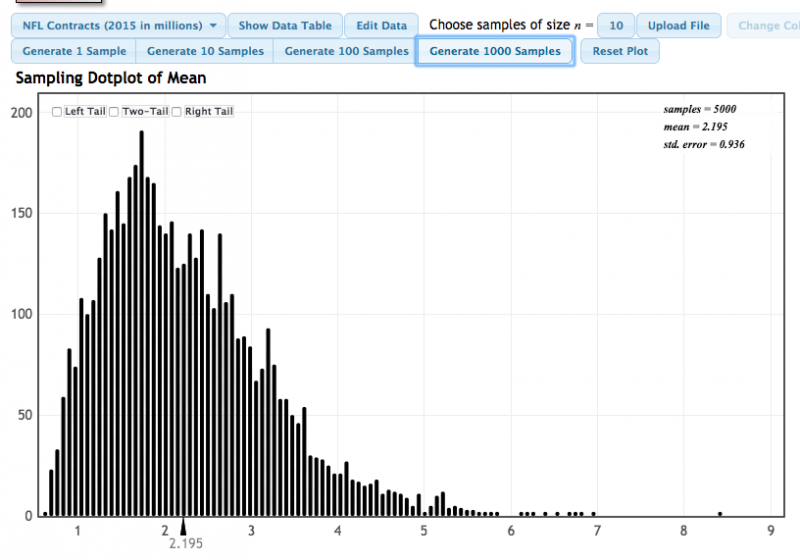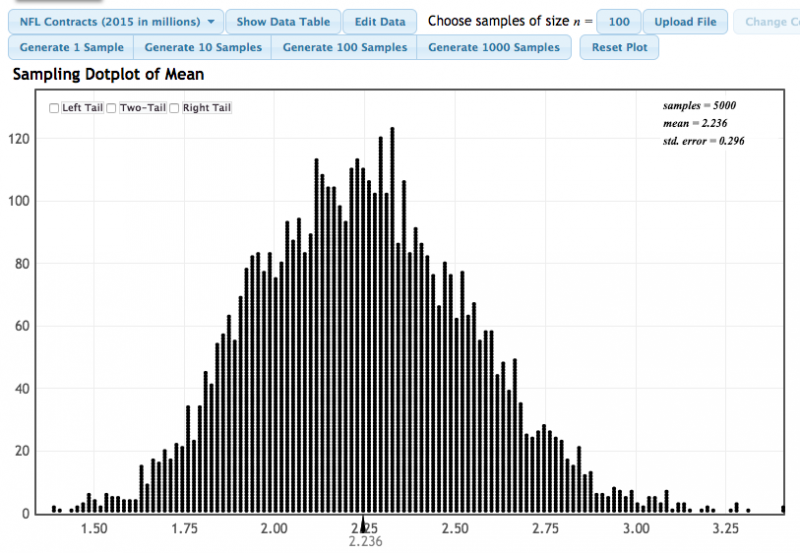# 4.1.3 - Impact of Sample Size

There is an inverse relationship between sample size and standard error.  In other words, as the sample size increases, the variability of sampling distribution decreases.

Also, as the sample size increases the shape of the sampling distribution becomes more similar to a normal distribution regardless of the shape of the population.

Example: Mean NFL Salary

The built-in dataset "NFL Contracts (2015 in millions)" was used to construct the two sampling distributions below. In the first, a sample size of 10 was used. In the second, a sample size of 100 was used.

#### Sample size of 10:#### Sample size of 100:With a sample size of 10, the standard error of the mean was 0.936. With a sample size of 100 the standard error of the mean was 0.296. When the sample size increased the standard error decreased.

Also know that the population was strongly skewed to the right. With the smaller sample size, the sampling distribution was also skewed to the right, though not as strongly skewed as the population. With the larger sample size, the sampling distribution was approximately normal.

## Example: Proportion of College Graduates Section

The built-in dataset "College Graduates" was used to construct the two sampling distributions below. In the first, a sample size of 10 was used. In the second, a sample size of 100 was used.

#### Sample size of 10:#### Sample size of 100:With a sample size of 10, the standard error of the mean was 0.143. With a sample size of 100 the standard error of the mean was 0.044. As the sample size increased the standard error decreased.

Also note how the shape of the sampling distribution changed. With the smaller sample size there were large gaps between each possible sample proportion. When the sample size increased, the gaps between the possible sampling proportions decreased. With the larger sampling size the sampling distribution approximates a normal distribution.